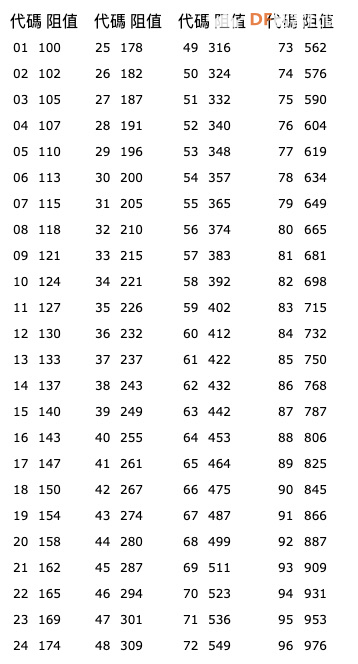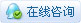# [求助问答] 什么是贴片电阻，贴片电阻阻值如何计算？# 什么是贴片电阻，贴片电阻阻值如何计算

SMD 电阻是一种设计为贴片安装的电阻器。这些SMD电阻器通常比传统的电阻器小得多，因此在电路板上占用的空间也小得多。SMD 电阻器通常只在专业制造的 PCB 中使用。

## 贴片电阻代码

### 例1 – 901

``````R = 90 x 10^1
R = 900``````

### 例2-3R4

``````R = 3R4
R = 3.4``````

### 例3–313

``````R = 31 x 10 ^ 3
R = 31,000``````

### 例4–R34

``````R = R34
R = .34``````

### 总结

• 三位都是纯数字的，前俩位不变，最后一位的数字表示的就是0的个数；

• 三位中带有“R”的，“R”的位置就是小数点的位置

## 如何计算四位数SMD代码的例子

### 例1 – 4402

``````R = 440 * 10^2
R = 44,000``````

### 例2-95R21

``````R = 95R21
R = 95.21``````

Code Multiplier
Z 0.001
Y / R 0.01
X / S 0.1
A 1
B / H 10
C 100
D 1,000
E 10,000
F 100,000

## EIA-96代码值表## EIA-96贴片电阻的计算实例

### 例2–11Y

``````R = 127 x 0.01
R = 1.27``````

### 例3-67C

``````R = 487 x 100
R = 48,700``````

### 问题来了

##### 97S代表的电阻值是多少呢？

B Color Image Link Quote Code Smilies |上传

[[wsData.name]]

#### 硬件清单

• [[d.name]]